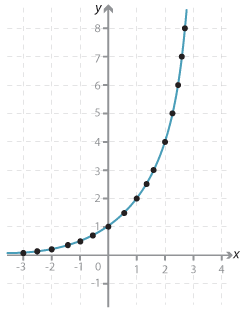#### Exponential graphs

The values of y = 2$$^x$$ for a number of values of x were obtained using a calculator, and are shown in the table below.
We can then plot the ordered pairs (x, 2$$^x$$) to produce a graph of y = 2$$^x$$.

 $$x$$ $$y$$ −3 −2.5 −2 −1.5 −1 −0.5 0 0.5 1 1.5 2 2.5 3 0.125 0.177 0.25 0.354 0.5 0.707 1 1.414 2 2.828 4 5.657 8Detailed description

Note that although we have 'joined the dots' to form a smooth curve, we have not given any meaning at this stage to 2$$^x$$ when it is an irrational number; thus we cannot deal with this problem at this stage.

We note the following features of the graph:

• The graph is increasing.
• The values increase quite rapidly as we move along the axis.
• On the left-hand side, the graph approaches, but never reaches, the axis.

### How much rice?

The old man had requested 36 893 488 147 419 103 230 grains of rice.